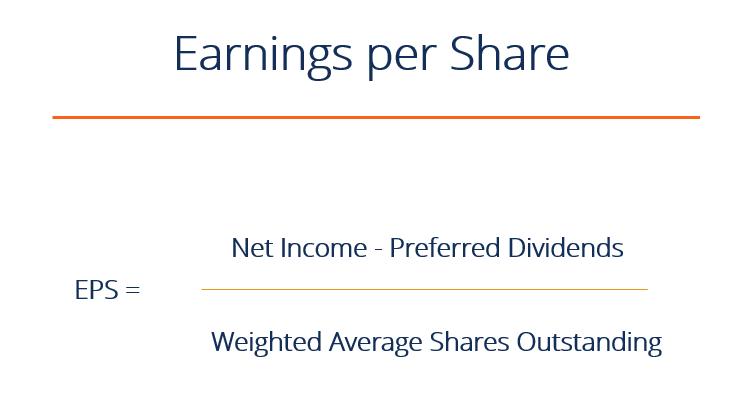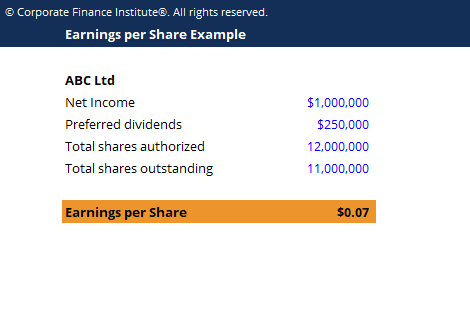# Earnings Per Share Formula (EPS)

Net income to common shareholders divided by shares outstanding

## What is the Earnings per Share (EPS) Formula?

EPS is a financial ratio, which divides net earnings available to common shareholders by the average outstanding shares over a certain period of time. The EPS formula indicates a company’s ability to produce net profits for common shareholders. This guide breaks down the Earnings per Share formula in detail.

A single EPS value for one company is somewhat arbitrary. The number is more valuable when analyzed against other companies in the industry, and when compared to the company’s share price (the P/E Ratio). Between two companies in the same industry with the same number of shares outstanding, higher EPS indicates better profitability. EPS is typically used in conjunction with a company’s share price to determine whether it is relatively “cheap” (low P/E ratio) or “expensive” (high P/E ratio).### Earnings per Share Formula

There are several ways to calculate earnings per share.

Below are two versions of the earnings per share formula:

EPS = (Net Income – Preferred Dividends) / End of period Shares Outstanding

EPS = (Net Income – Preferred Dividends) / Weighted Average Shares Outstanding

The first formula uses total outstanding shares to calculate EPS, but in practice, analysts may use the weighted average shares outstanding when calculating the denominator. Since outstanding shares can change over time, analysts often use last period shares outstanding.

There is also often talk of diluted EPS in financial reports. Diluted EPS includes options, convertible securities, and warrants outstanding that can affect total shares outstanding when exercised.

Another type of earnings per share formula is adjusted EPS. This removes all non-core profits and losses, as well as those in minority interests. The focus of this calculation is to see only profit or loss generated from core operations on a normalized basis.

### Earnings Per Share Formula Example

ABC Ltd has a net income of \$1 million in the third quarter. The company announces dividends of \$250,000. Total shares issued for the period was 12,000,000, with total shares outstanding at 11,000,000.

The EPS of ABC Ltd. would be:

EPS = (\$1,000,000 – \$250,000) / 11,000,000
EPS = \$0.068

Since every share receives an equal slice of the pie of net income, they would each receive \$0.068.

### Earnings per Share Formula Template

As you can see in the Excel screenshot below, if ABC Ltd has a net income of \$1 million, dividends of \$0.25 million, and shares outstanding of 11 million, the earnings per share formula is (\$1 – \$0.25) / 11 = \$0.07.

Note that many companies do not have preferred shares, and for those companies, there are no preferred dividends that need to be deducted. The reason preferred dividends are deducted is that EPS represents only the earnings available to common shareholders, and preferred dividends need to be paid out before common shareholders receive anything.### Video Explanation of Earnings Per Share Formula (EPS)

Watch this short video to quickly understand the main concepts covered in this guide, including what Earnings Per Share is, the formula for EPS, and an example of EPS calculation.

### Price to Earnings Ratio

Earnings per share are almost always analyzed relative to a company’s share price. This ratio is known as the Price to Earnings Ratio (or P/E ratio).  Learn more in CFI’s guide to the Price-Earnings Ratio.

This has been CFI’s guide to the earnings per share formula.  To learn more about other forms of financial analysis and to advance your career as a certified financial analyst, explore these additional resources below:

• Cash EPS
• Price-Earnings Ratio
• Analysis of Financial Statements
• Financial Modeling Guide

### Financial Analyst Training

Get world-class financial training with CFI’s online certified financial analyst training program!

Gain the confidence you need to move up the ladder in a high powered corporate finance career path.

Learn financial modeling and valuation in Excel the easy way, with step-by-step training.